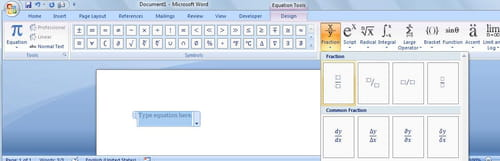2
Thanks

A few words of thanks would be greatly appreciated.

# Word - Insert Mathematical equation,formulas• Word 2007 comes with an handy feature that allows you to insert mathematical equation
• To insert a formula in a Word document.
• Click to place your cursor where you want the symbols to be entered in the document,
• Select the Insert Menu>Equation button> select one of the predefine formula or click on the "Insert New Equation" to write down your own one.2
Thanks

A few words of thanks would be greatly appreciated.